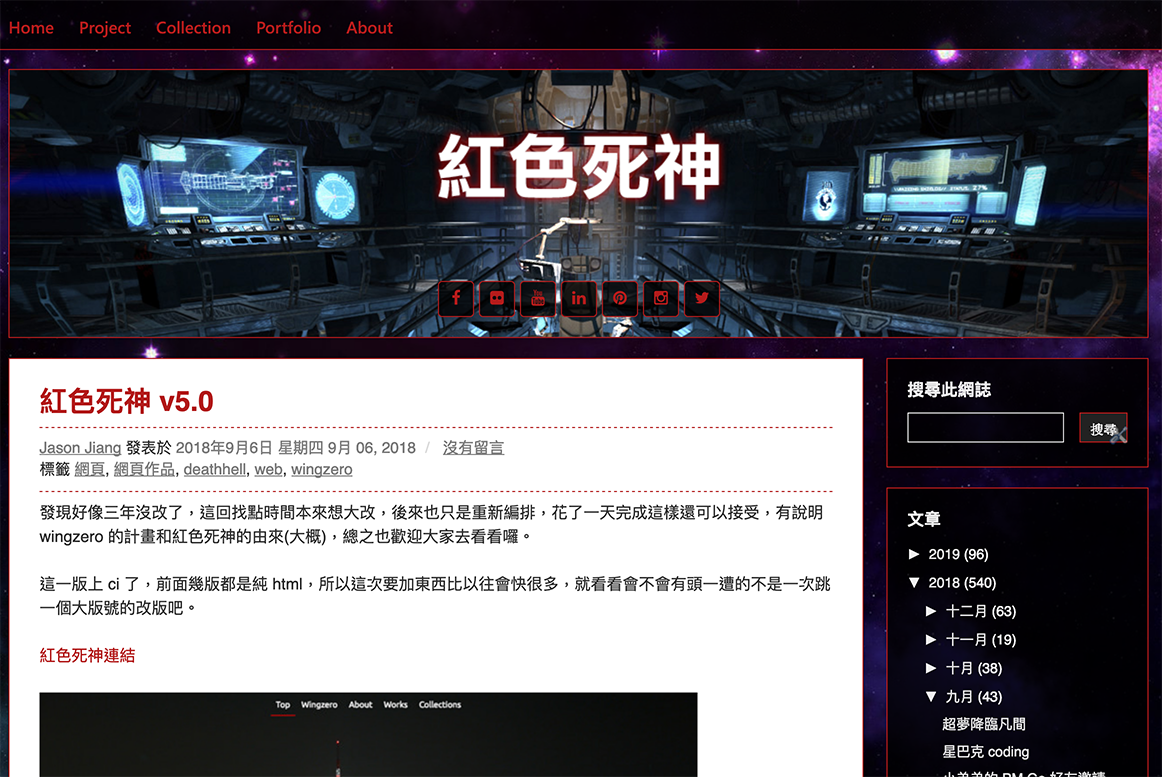# 紅色死神網誌和無名紅色死神改版# 用 js 把數字加千分位的方法

```function toThousands(num) {
var num = (num || 0).toString(), result = '';
while (num.length > 3) {
result = ',' + num.slice(-3) + result;
num = num.slice(0, num.length - 3);
}
if (num) { result = num + result; }
return result;
}
```

# Pokémon GO AR 照上新聞# Pokemon GO 寵物小精靈 AR 影相 GO Snapshot　識玩原來好搞笑

Pokemon GO 寵物小精靈 AR 影相 GO Snapshot　識玩原來好搞笑

Pokemon GO 加入了 AR 影相功能 GO Snapshot，不過一般玩家未必有太多時間研究新功能的玩法。台灣的 Pokemon GO 資深玩家，Pokemon-info網站的站主「紅色死神」江舜智，就以 GO Snapshot 拍攝了不少非常搞笑的小精靈照片，在網站刊登跟網友分享。

# 笑死！寶可夢惡搞AR照這樣拍　網友：真的很會# 解決背景使用 SVG 會自動變得跟內容一樣高的問題# 替你的 Blogger 加上圖片 lazyload 效果

Lazyload 是許多網站都會加上避免網頁開啟時一次讀取太多圖片的效果，在 WP 上有 plugin 可以安裝使用，要在 blogger 上使用的話相較就沒有那麼簡單。

```//c.failurelimit){return false}}});var f=a.grep(d,function(a){return!a.loaded});d=a(f)})}this.each(function(){var b=this;if(undefined==a(b).attr("original")){a(b).attr("original",a(b).attr("src"))}if("scroll"!=c.event||undefined==a(b).attr("src")||c.placeholder==a(b).attr("src")||a.abovethetop(b,c)||a.leftofbegin(b,c)||a.belowthefold(b,c)||a.rightoffold(b,c)){if(c.placeholder){a(b).attr("src",c.placeholder)}else{a(b).removeAttr("src")}b.loaded=false}else{b.loaded=true}a(b).one("appear",function(){if(!this.loaded){a("").bind("load",function(){a(b).hide().attr("src",a(b).attr("original"))[c.effect](c.effectspeed);b.loaded=true}).attr("src",a(b).attr("original"))}});if("scroll"!=c.event){a(b).bind(c.event,function(c){if(!b.loaded){a(b).trigger("appear")}})}});a(c.container).trigger(c.event);return this};a.belowthefold=function(b,c){if(c.container===undefined||c.container===window){var d=a(window).height()+a(window).scrollTop()}else{var d=a(c.container).offset().top+a(c.container).height()}return d<=a(b).offset().top-c.threshold};a.rightoffold=function(b,c){if(c.container===undefined||c.container===window){var d=a(window).width()+a(window).scrollLeft()}else{var d=a(c.container).offset().left+a(c.container).width()}return d<=a(b).offset().left-c.threshold};a.abovethetop=function(b,c){if(c.container===undefined||c.container===window){var d=a(window).scrollTop()}else{var d=a(c.container).offset().top}return d>=a(b).offset().top+c.threshold+a(b).height()};a.leftofbegin=function(b,c){if(c.container===undefined||c.container===window){var d=a(window).scrollLeft()}else{var d=a(c.container).offset().left}return d>=a(b).offset().left+c.threshold+a(b).width()};a.extend(a.expr[":"],{"below-the-fold":"\$.belowthefold(a, {threshold : 0, container: window})","above-the-fold":"!\$.belowthefold(a, {threshold : 0, container: window})","right-of-fold":"\$.rightoffold(a, {threshold : 0, container: window})","left-of-fold":"!\$.rightoffold(a, {threshold : 0, container: window})"})})(jQuery);\$(function(){\$("img").lazyload({placeholder:"你的讀取動畫網址",effect:"fadeIn",threshold:"-50"})})//]]>
```